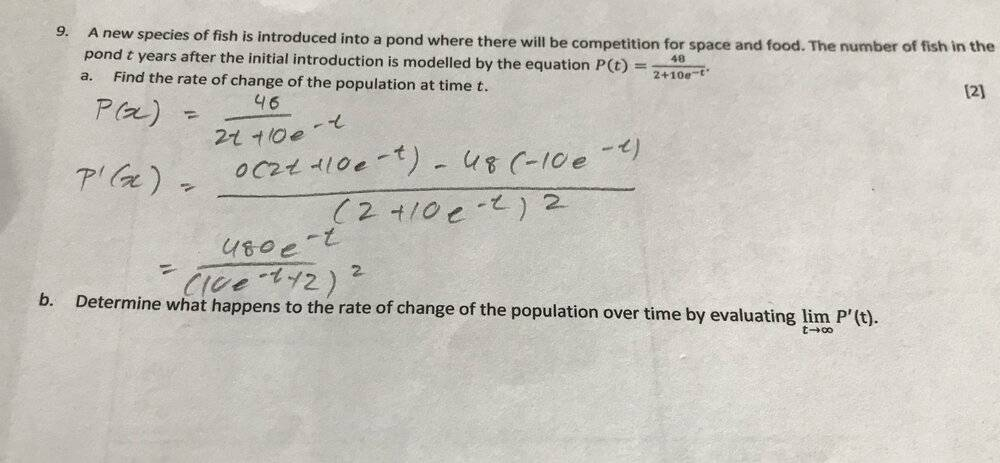# Determine what happens to the rate of change of the population over time

Homework Statement:
I'm not sure how to start question b.) I understand that I have the denominator powered to the square, it's function "grows faster" than the function in the numerator.
Relevant Equations:
n/aharuspex
Homework Helper
Gold Member
2020 Award
it's function "grows faster" than the function in the numerator.
Does it grow? What happens to e-t as t increases?
Btw, you have a sign error in part a. Correction: no I had a sign error.

Last edited:
epenguin
Homework Helper
Gold Member
I am not seeing this sign mistake and think your answer is correct. However it was unnecessary to use the full formula for derivative of u(x)/v(x) - since u is just a constant you only needed that for 1/v(x).

You are not thinking about the rest in quite the right way. What happens to e-t as t increases and as it becomes very large? Alternatively you get something that might be mere self-evident to you if you divide top and bottom of the fraction by e-t.

I am not seeing this sign mistake and think your answer is correct. However it was unnecessary to use the full formula for derivative of u(x)/v(x) - since u is just a constant you only needed that for 1/v(x).

You are not thinking about the rest in quite the right way. What happens to e-t as t increases and as it becomes very large? Alternatively you get something that might be mere self-evident to you if you divide top and bottom of the fraction by e-t.
So you'll have lim t---> infinite , the function in the denominator will grow faster, so as t grows, P'(x) approaches zero. I believe another way to see this is to note that for t---> inf, P(x)---> 24, therefore reaching a constant value, with zero rate of change. My rate would be zero, right?

epenguin
Homework Helper
Gold Member
Not very convincing, in fact wrong. Firstly, rather than the denominator, what about the numerator??

Last edited:
haruspex
Homework Helper
Gold Member
2020 Award
I am not seeing this sign mistake
You are right - my mistake.
the function in the denominator will grow faster,
I refer you again to my question in post #2.

Mark44
Mentor
The work in post #1 has an error.
You (@ttpp1124) have ##P'(x) = \frac{0(2t + 10e^{-t}) - 48(-10e^{-t})}{(2 + 10e^{-t})^2}##.
The error is in the 2nd term in the numerator.
What's the derivative with respect to t, of ##2t + 10e^{-t}##?

Also, both P and P' are functions of time t, not x.

Edit: The type in the problem statement is so small that I didn't notice that ##\frac{48}{2 + 10e^{-t}}## there had turned into ##\frac{48}{2t + 10e^{-t}}## in the written work.

Last edited:
benorin
Homework Helper
There is a typo/error in the denominator of ##P(t)##, an extra ##t##, not that it matters much.

•Mark44